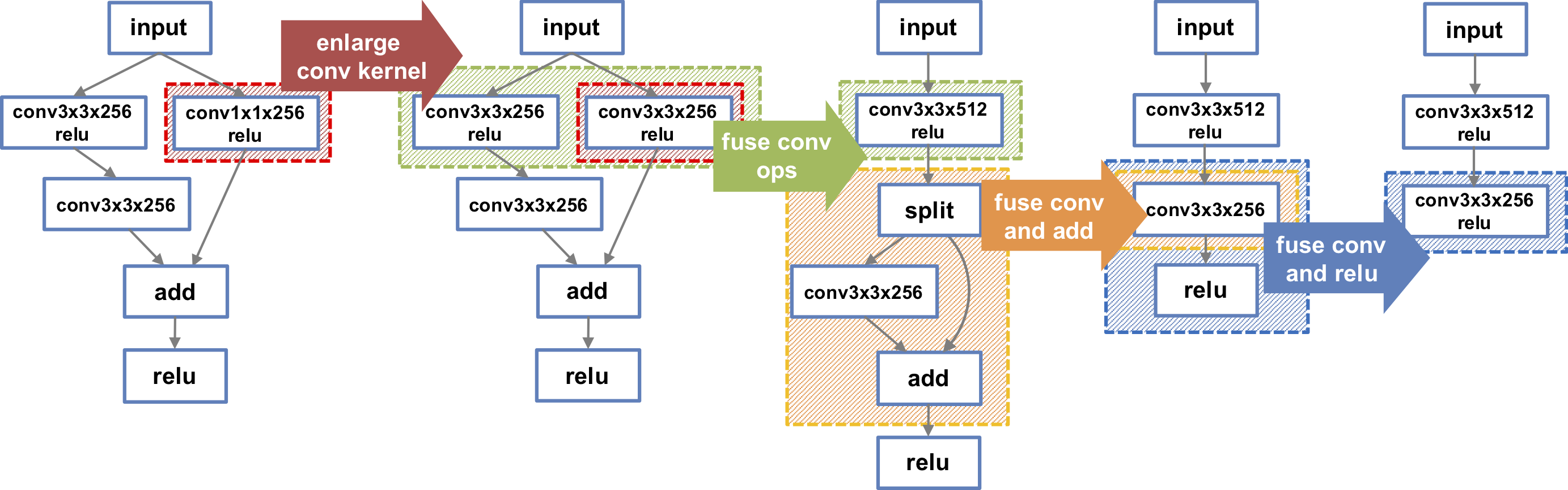# TASO

TASO optimizes the computation graphs of DNN models using automatically generated and verified graph transformations. For an arbitrary DNN model, TASO uses the auto-generated graph transformations to build a large search space of potential computation graphs that are equivalent to the original DNN model. TASO employs a cost-based search algorithm to explore the space, and automatically discovers highly optimized computation graphs. TASO outperforms the graph optimizers in existing deep learning frameworks by up to 3x.TASO directly optimizes any pre-trained DNN models in ONNX, TensorFlow, and PyTorch graph formats. TASO also provides a Python interface for optimizing arbitrary DNN architectures. TASO supports exporting the optimized computation graphs to ONNX, which can be directly used as inputs by most existing deep learning frameworks.

For example, the following figures shows how TASO automatically performs a series of non-trivial transformations and improves the inference performance of a ResNet module by 1.3x on a V100 GPU.